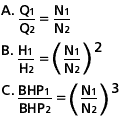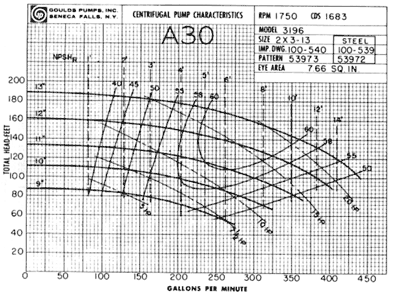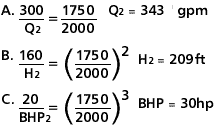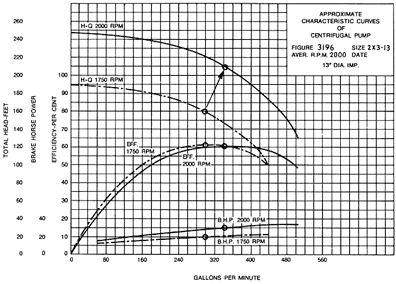### AffinityLaws

The affinity laws express the mathematical relationship between the several variables involved in pump performance. They apply to all types of centrifugal and axial flow pumps. They are as follows:

1. With impeller diameter D held constant:
Where:
Q = Capacity, GPM
BHP = Brake Horsepower
N = Pump Speed, RPM2. With speed N held constant:When the performance (Q1, H1, & BHP1) is known at some particular speed (N1) or diameter (D1), the formulas can be used to estimate the performance (Q2, H2, & BHP2) at some other speed (N2) or diameter (D2). The efficiency remains nearly constant for speed changes and for small changes in impeller diameter.

Example:
To illustrate the use of these laws, refer to Fig. 8 below. It shows the performance of a particular pump at 1750 RPM with various impeller diameters. This performance data has been determined by actual tests by the manufacturer. Now assume that you have a 13" maximum diameter impeller, but you want to belt drive the pump at 2000 RPM.Fig. 8 Composite Performance Curve

The affinity laws listed under 1 above will be used to determine the new performance, with N1 1750 RPM and N2 = 2000 RPM. The first step is to read the capacity, head, and horsepower at several points on the 13" dia. curve in Fig. 9 below. For example, one point may be near the best efficiency point where the capacity is 300 GPM, the head is 160 ft, and the BHP is approx. 20 hp.This will then be the best efficiency point on the new 2000 RPM curve. By performing the same calculations for several other points on the 1750 RPM curve, a new curve can be drawn which will approximate the pump's performance at 2000 RPM, Fig. 9.

Trial and error would be required to solve this problem in reverse. In other words, assume you want to determine the speed required to make a rating of 343 GPM at a head of 209 ft. You would begin by selecting a trial speed and applying the affinity laws to convert the desired rating to the corresponding rating at 1750 RPM. When you arrive at the correct speed, 2000 RPM in this case, the corresponding 1750 RPM rating will fall on the 13" diameter curve.Fig. 9